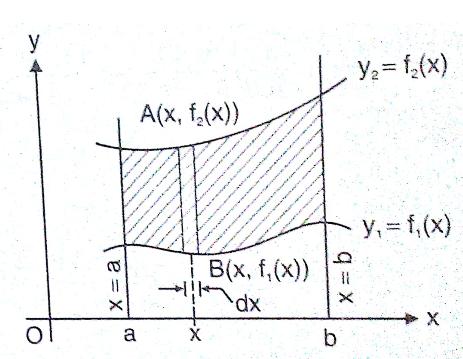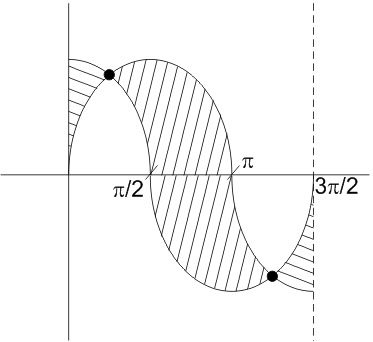# The area bounded by the curvesandbetween the ordinatesOption 1)Option 2)Option 3)Option 4)As we learnt in

Area along x axis -

Letbe two curve then area bounded between the curves and the lines

x = a and x = b is- whereinWhereOption 1)This option is correct

Option 2)This option is incorrect

Option 3)This option is incorrect

Option 4)This option is incorrect